Author: Oscar Cronquist Article last updated on March 14, 2022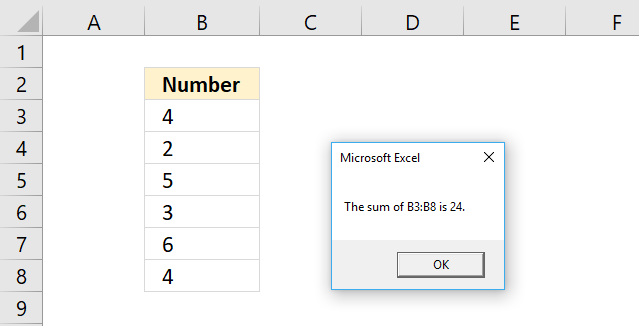## 1. FOR NEXT Syntax

For counter = start To end Step step

... statements ...

[ Exit For ]

... statements ...

Next [ counter ]

counter is a variable
start, end, and step are numbers

## 2. FOR NEXT with a counter variable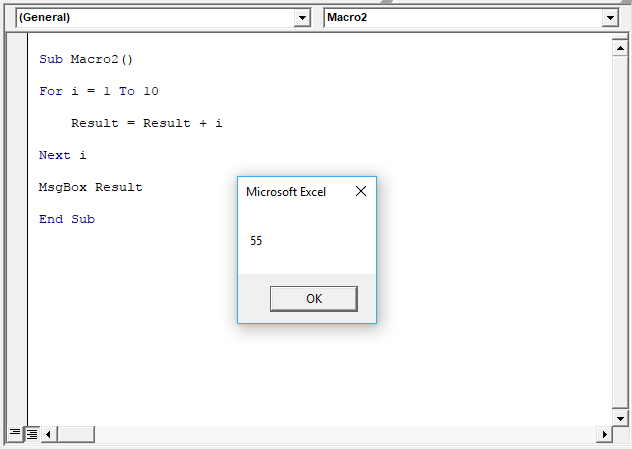You can have a variable that counts for you, in this case, it is variable i. The FOR NEXT statement runs the lines between FOR and NEXT ten times incrementing variable i with 1 each time. See picture above.

It starts with 1 and continues up to 10. 1+2+3+4+5+6+7+8+9+10 = 55. It is not necessary to include the variable name after the NEXT statement, however, it will be easier to read your code if you do so.

The message box then displays the sum. As soon as you press with left mouse button on the OK button the macro ends.

```

'Name macro
Sub Macro1()

'For Next statement
For i = 1 to 10

'Add number stored in variable i to variable Result
Result = Result + i

'Continue with next number
Next i

'Display message box containing number stored in variable Result
MsgBox Result

'Exit macro
End Sub
```

## 3. FOR each NEXT exampleThe picture above demonstrates a FOR NEXT statement that goes through each cell value in cell range B3:B8 and adds it to a total.

The Message box then displays the sum. 4+2+5+3+6+4 is 24. Here is the subroutine:

```'Name macro
Sub Macro1()

'For each Next statement
For Each Value In Range("B3:B8")

Result = Result + Value

'Continue with next value in cell range
Next Value

'Display message box containing number in variable result
MsgBox Result

'Exit macro
End Sub
```

The Value variable stores the number from cell B3 in the first iteration then continues to B4 and overwrites the previous value, and so on. The Result variable adds each value in every iteration and builds a sum.

The macro repeats the lines between FOR and NEXT as many times as there are cells in Range("B3:B8).

The message box displays the sum and you then need to press with left mouse button on the OK button to proceed.

## 4. FOR NEXT with counter variable and STEP keyword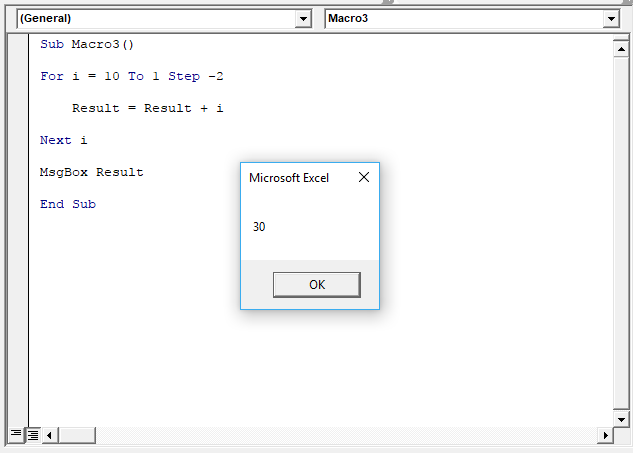The STEP keyword allows you to increase or decrease the counter value with a number you specify.

If you use a negative step number make sure the end counter number is less than the start counter number to avoid your macro being in an endless loop.

In the above example, the counter variable is i and it starts with 10 and ends with 1, the STEP value is -2. 10+8+6+4+2=30.

```
'Name macro
Sub Macro1()

'For each Next statement
For i = 10 To 1 Step -2

Result = Result + i

'Continue with next value in cell range
Next i

'Display message box containing number in variable result
MsgBox Result

'Exit macro
End Sub ```

## 5. FOR NEXT and EXIT FOR statements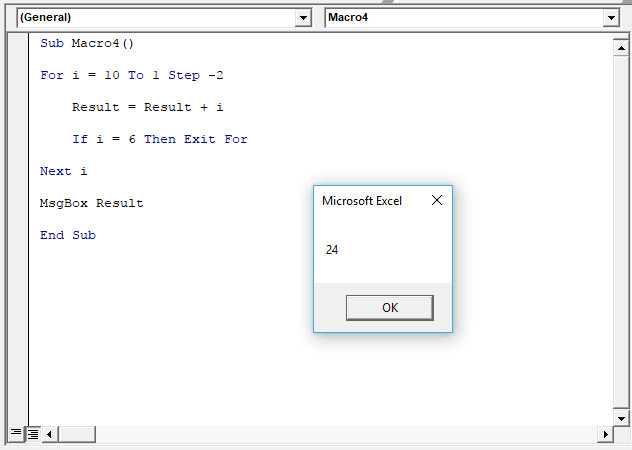The EXIT FOR statement allows you to stop iterating through a FOR NEXT statement which is handy if, for example, an error value shows up.

The picture above uses the IF THEN statement to check if counter variable i is equal to 6. 10 + 8 + 6 = 24.

```

'Name macro
Sub Macro1()

'For each Next statement
For i = 10 To 1 Step -2

Result = Result + i

'Check if variable i equals 6 and Exit For Next statement if true
If i = 6 then Exit For

'Continue with next value in cell range
Next i

'Display message box containing number in variable result
MsgBox Result

'Exit macro
End Sub
```

## 6. FOR each NEXT - array values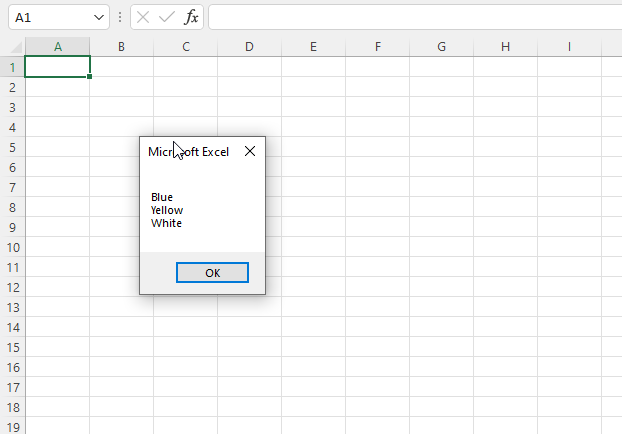The code below demonstrates a FOR NEXT statement that goes through each value in an array variable.

The Message box then displays each array value on a new line. Here is the subroutine:

```'Name macro
Sub Macro1()

'Save values to an array variable
arr = Array("Blue", "Yellow", "White")
'For each Next statement
For i = LBound(arr) To UBound(arr)

'Concatenate array value to variable txt and a new line
res = res & arr(i) & vbNewLine

'Continue with next value in array
Next i

'Display message box containing number in variable res
MsgBox res

'Exit macro
End Sub
```